# Colpitts Oscillator

Published  February 21, 2019   0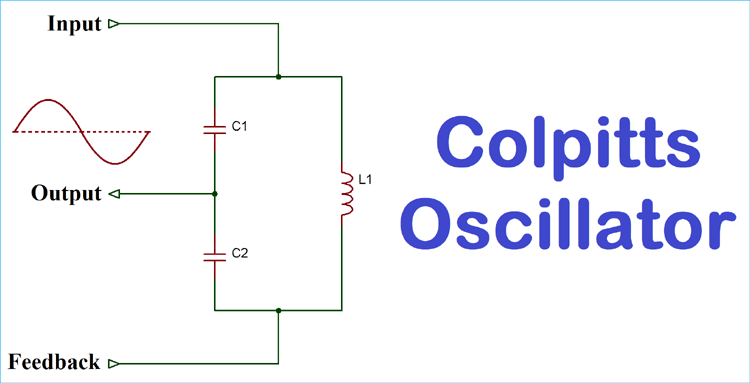An oscillator is a mechanical or electronic construction which produces oscillation depending on few variables. We all have devices which need oscillators like a traditional clock or a wristwatch. Various types of metal detectors, computers where microcontroller and microprocessors are involved use oscillators, especially electronics oscillator which produces periodic signals. We discussed few oscillators in our previous tutorials:

The Colpitts oscillator was invented by American engineer Edwin H. Colpitts in 1918. Colpitts oscillator works with a combination of inductors and capacitors by forming an LC filter. Same as other oscillators Colpitts oscillator consists of a gain device, and the output is connected with an LC circuit feedback loop. The Colpitts oscillator is a linear oscillator which produces a sinusoidal waveform.

### The Tank Circuit

The main oscillation device in Colpitts oscillator is created using the tank circuit. The tank circuit consists of three components- a inductor and two capacitors. Two capacitors are connected in series, and these capacitors are further connected in parallel with inductor.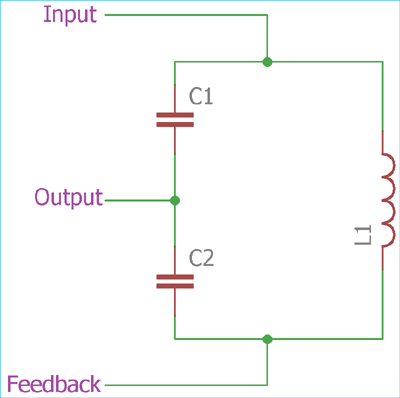In the above image, three components of the tank circuit are shown with proper connections. The process starts with charging of two capacitors C1 and C2. Then inside the tank circuit, these two series capacitors discharge into the parallel inductor L1 and the stored energy in the capacitor transferred to the inductor. Due to the capacitor connected in parallel, the inductor now discharged by the two capacitors and the capacitors start to charge again. These charging and discharging in both of the components continues and thus providing an oscillation signal across it.

The oscillation is highly depended on the capacitors and the inductor’s value. Below formula is to determine the oscillation frequency:

```F = 1 / 2π√LC
```

where F is frequency and L is Inductor, C is the total equivalent capacitance.

The equivalent capacitance of the two capacitors can be determined using

`C = (C1 x C2) / (C1 + C2)`

During this oscillation phase in the tank circuit, some energy loss is occurred. To compensate this lost energy and to sustain the oscillation inside the tank circuit, a gain device is required. There are many different types of gain devices are used to compensate the loss of energy inside the tank circuit. The most common gain devices are transistors and operational amplifiers.

### Transistors Based Colpitts Oscillator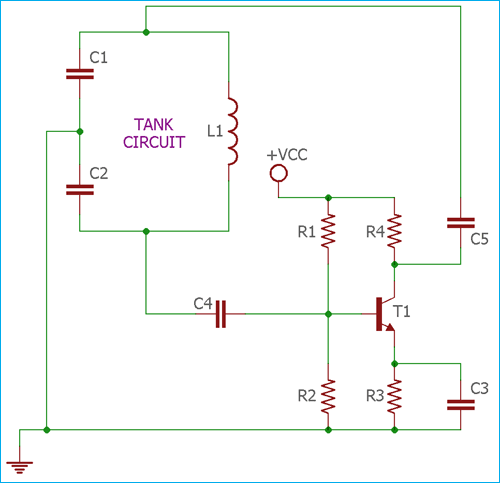In the above image, the Transistor based Colpitts Oscillator is shown where the main gain device of the oscillator is an NPN transistor T1.

In the circuit, resistor R1 and R2 are required for the base voltage. These two resistors are used to make a voltage divider across Transistor T1’s base. Resistor R3 is used as an emitter resistor. This resistor is very useful to stable the gain device during the thermal drift. The capacitor C3 is used as an emitter bypass capacitor which is connected in parallel with the resistor R3. If we remove this C3 capacitor, the amplified AC signal will be dumped across resistor R3 and results in a poor gain. So, the capacitor C3 is provided an easy path for the amplified signal. The feedback from the tank circuit is further connected using the C4 to the transistor T1's base.

The oscillation of the transistor based Colpitts oscillator circuit is depended on the phase shift. This is well known as barkhausen criterion for the oscillator. As per the Barkhausen Criterion, the loop gain should be slightly greater than the unity and the phase shift around the loop needs to be 360 degrees or 0 degrees. So, during this case, to provide the oscillation across the output, the total circuit needs 0 degrees or 360-degree phase shift. The transistor configuration as common emitter provides 180-degree phase shift whereas the tank circuit also contributes an additional 180-degree phase shift. By combining this two-phase shifts the total circuitry achieves 360-degree phase shift which is responsible for the oscillation.

The feedback can be controlled using the two capacitors C1 and C2. These two capacitors are connected in series and the junction is further connected with the supply ground. The voltage across C1 is much greater than the voltage across C2. By changing those two capacitor values we can control the feedback voltage which is further fed back to the tank circuit. The determination of the feedback voltage is a crucial part of the circuitry because the low amount of feedback voltage would not activate the oscillation whereas a high amount of feedback voltage will end up in destroying the output sine wave and induce distortion.

The Colpitts oscillator can be tuned by changing the value of inductance and capacitance. There are two ways to make the Colpitts oscillator work in a variable tuning configuration.

The first way is to change the inductor as a variable inductor and the other way is to change the capacitors as a variable capacitor. In the second option, as the feedback voltage is highly dependable on the ratio of C1 and C2 it is advisable to use a simple gang. So that when there is variation in one capacitor the other capacitor also changes its capacitance in accordance with it.

### Op-Amp Based Colpitts Oscillator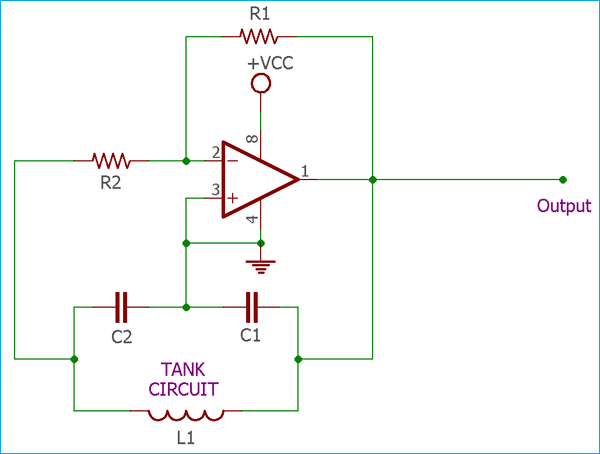In the above image op-amp based Colpitts oscillator circuit is shown. The operational amplifier is in inverting configuration mode. Resistors R1 and R2 are used due to provide the necessary feedback to the operational amplifier. The tank circuit is connected along with the single inductor in parallel with two series capacitors. The input of the operational amplifier is connected to the feedback of the tank circuit.

The working is the same as discussed in the above transistor based Colpitts oscillator circuit. During startup, the op-amp amplifies noise signal which is responsible to charge two capacitors. The gain of Op-amp based Colpitts Oscillator is higher than the Transistor based Colpitts Oscillator.

### Difference between Colpitts Oscillator and Hartley Oscillator

The Colpitts oscillator is very similar to Hartley oscillator but there is a difference in construction between these two. Although these two oscillator circuit consist three components as a tank circuit but the Colpitts oscillator uses a single inductor in parallel with two capacitors in series whereas the Hartley oscillator uses exactly opposite, one single capacitor in parallel with two inductors in series. Colpitts oscillator performs more stable in high-frequency operation than the Hartley Oscillator.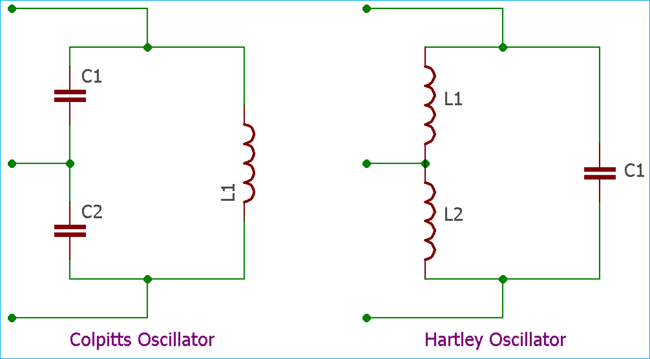The Colpitts oscillator is an excellent choice in high-frequency operation. It can produce output frequency in Megahertz range as well as in Kilohertz range.

### Application of Colpitts Oscillator Circuit

1. Due to the difficulties in a smooth variation of inductor and capacitor, the Colpitts oscillator is mainly used for fixed frequency generation.

2. The main use of Colpitts oscillator is in mobile or other radio frequency controlled communications devices.

3. In high-frequency oscillation, Colpitts oscillator is an excellent choice. Thus high-frequency oscillator based devices use Colpitts Oscillator.

4. In a few applications where continuous and undamped oscillation is needed in addition with thermal stability, Colpitts Oscillator is used.

5. For those applications which need a wide range of frequencies with minimum noise induced.

6. Many types of SAW-based sensors use Colpitts oscillator

7. Various types of metal detector use the Colpitts oscillator.

8. Frequency modulation related radio frequency transmitter use Colpitts oscillator.

9. It has a huge application in military and commercial grade products.

10. In microwave applications, signal masking related chaotic circuits is also required Colpitts oscillator in the different frequency range.